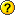Tabla de Contenidos

# The force of gravity "g"

## What is a force?

Force, is all that can cause:

• Putting a body in motion (moving a ball giving it a kick)
• Stop a body (a goalkeeper who catches the ball)
• Distort a body (block of dough into dummy)
• Divert a body (the goalkeeper doesn´t catches the ball, but it changes its course to check that you are not a goal)

## What´s the force of gravity?

When a body left suspended in the air and does not exert any force on it, the body falls vertically to the ground.
This is due to the terrestrial gravitational force, ie, the force of attraction exerted by the earth towards its center, over the bodies that are in its vicinity.

## How does "g"?

We use two laws of the dynamics:

1. RULE OF UNIVERSAL GRAVITATION NEWTON:

“The intensity of the force that attracts two bodies depends on their masses and the distance between them”.

That:2. BASIC LAW OF THE DYNAMICS

“The force exerted on a body is directly proportional to the aceleration to be announced”.

That:Galileo Galilei proved that all bodies which are on earth, when released, fall with the same acceleration regardless of their mass since the fall of a body depends on the strenght of gravity.

That:Equating the two previous findings:=Thus we see that the gravity change if R varies with latitude an altitude.### Example

What is the variation of "g" at o point at sea level and in the Himalayas?

The information that we know are:

• M = mass of Earth =• R = radius of Earth(at sea level) =• G = universal gravitational constant =Substituting these values into the formula we have obtained, from meters to kilometers:

• At a point at sea level, the force of gravity is:=• At one point in the Himalaya (highest mountain on Earth, located in Asia which consists of mountain exceeding 8000 meters in altitude), the force of gravity is:

Now, the radius is: R=6.378 + 0.08=6.386 km and is:=So, we can conclude that: the force of gravity decreases as we move away from the center of the Earth.

force_of_gravity_g.txt · Última modificación: 24/04/2017 13:13 (editor externo)